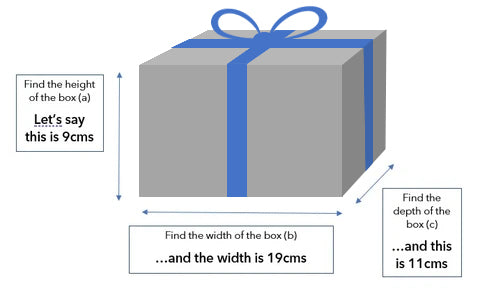Posted on by Jeannette Jones### This is a simple calculation that will save you the annoyance of under or over buying on Ribbon.

First, find the size of the box you are wrapping

• Find the height of the box (a)
• Find the width of the box (b)
• Find the depth of the box (c)

If you are measuring in inches you will need to take your calculation and convert to centimetres (x2.5) as Ribbon usually comes by the metre (1 metre = 100centimetres).Now think about where you would like the ribbon to go around the box

For this demonstration we're going to wrap like the diagram, with ribbon on all 6 sides of the box.  However, if you are only going around 2 sides then you'll need to add the sum of those sides together (and be aware that it's double each side).

• The ribbon will go down the height of the box 4 times - so take the height measurement and times by 4
• The ribbon will go across the width of the box twice - so take the width measurement and times by 2
• The ribbon will go across the depth of the box twice - so take the depth measurement and times by 2This will give you the amount of ribbon needed for the sides of the box.

In our example above we have,

• height of 9cms x 4 = 36cms
• width of 19cms x 2 = 38cms
• depth of 11cms x 2 = 22cms

The total amount of ribbon for the sides (36 + 38 + 22) is therefore 96cms

Don't forget about the Bow on top.

Take a ball of string and tie a bow the size you would like the real one to be.

Now untie the bow and measure how much string you've used.

You'll need to include this.

This will give you a total measurement of ribbon needed per box including the bow.  You may like to add a small margin for error, but you should be near on a very accurate estimation for the Ribbon per box.

Remember this calculation is in centimetres and most ribbon is sold in metres (1 metre = 100centimetres).

So for my example above, after using a ball of string to make a bow, I find that we need an additional 24cms to make a bow.

Making a total of 120cms per box (96cm + 24cm).

Now multiple this amount by the number of boxes you'll be wrapping to find how many centimetres of ribbon you'll need.  (This figure might sound very very big, but it is still in centimetres!)

Compare it now to the amount of Ribbon that is on 1 roll, to see how many rolls of ribbon you'll need.

For my example of 120cms per box, that is 1.2metres - Compare this with 1 roll of 25metres of Ribbon.

25 divided by 1.2 is 20.8, so 1 roll will wrap 20 boxes of the same size.

Consider the wastage

How many items will you wrap with 1 roll of Ribbon, will the rest of the roll of Ribbon be wasted?  Would a bigger roll of Ribbon mean less wastage.  Depending on how frequent a user you are, there maybe ways to reduce the waste and save you roll changes.  (If you are wrapping a range of sizes of box, or very small boxes, the wastage is less.)

### We hope this guide helps you.  If you need more help, or have more tips to share on measuring ribbon, please contact the Kudos team 01923 220055.